# Uses Of Differentiation

### Summary

• If y = f(x) is an increasing function then$\frac { dy }{ dx } \quad >\quad 0$
• If y = f(x) is a decreasing function then$\frac { dy }{ dx } \quad <\quad 0$
• Stationary Points are obtained by solving the equation$\frac { dy }{ dx } \quad =\quad 0$
• 3 types of stationary points:

i) Maximum Point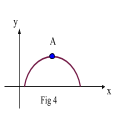ii) Minimum Point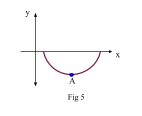iii) Point of Inflexion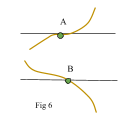#### Increasing Function

Refer to Fig 1, consider a point A on the curve y = f(x). A tangent is drawn at A and the gradient of the tangent at A is$\frac { dy }{ dx }$.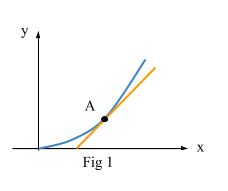In this situation when x increases the function f(x) also increases so$\frac { dy }{ dx }$ positive. Hence, it is known as an increasing function.

If y = f(x) is an increasing function then$\frac { dy }{ dx } \quad >\quad 0$.

#### Decreasing Function

Refer to Fig 2, A tangent is drawn at A and the gradient of the tangent at A is$\frac { dy }{ dx }$.In this situation when x increases the function f(x) decreases so$\frac { dy }{ dx }$  is negative. Hence, it is known as a decreasing function.

If y = f(x) is a decreasing function then$\frac { dy }{ dx } \quad <\quad 0$.

#### Example #1

Q. Find the range of values of x such that$f\left( x \right) \quad =\quad 3{ x }^{ 2 }\quad +\quad 4x\quad -\quad 7$  is an increasing function.

Solution:$y\quad =\quad 3{ x }^{ 2 }\quad +\quad 4x\quad -\quad 7$$\frac { dy }{ dx } \quad =\quad 6x\quad +\quad 4$

As f(x) is an increasing function hence$\frac { dy }{ dx } \quad >\quad 0$.

So,$6x\quad +\quad 4\quad >\quad 0$$x\quad >\quad \frac { -2 }{ 3 }$       Ans

#### Stationary Points

Refer to Fig 3, consider a graph of y =  f(x). Notice the 4 main situations in the diagram.i) Along AB, gradient of the curve is increasing, therefore$\frac { dy }{ dx } \quad >\quad 0$ .
ii) On reaching point B, the tangent at B becomes parallel to the x axis. Hence, at B$\frac { dy }{ dx } \quad =\quad 0$.
iii) After passing B, the gradient of the curve becomes negative, therefore$\frac { dy }{ dx } \quad <\quad 0$ .
iv) As the curve now reaches C,$\frac { dy }{ dx }$  at C is again 0.

To conclude, point B and C are special points where the tangents being parallel to the x axis,$\frac { dy }{ dx }$ is 0.

Stationary Points are obtained by solving the equation$\frac { dy }{ dx } \quad =\quad 0$

#### Example #2

Q. Find the stationary values of$\quad f\left( x \right) \quad =\quad 4{ x }^{ 3 }\quad -\quad 3x\quad +\quad 12$

Solution:$\quad f\left( x \right) \quad =\quad 4{ x }^{ 3 }\quad -\quad 3x\quad +\quad 12$

Find$\frac { dy }{ dx }$$\frac { dy }{ dx } \quad =\quad f^{ ' }\left( x \right) \quad =\quad 12{ x }^{ 2 }\quad -\quad 3$

We know that at stationary points$\frac { dy }{ dx } \quad =\quad 0$.

Hence:$12{ x }^{ 2 }\quad -\quad 3\quad =\quad 0$${ x }^{ 2 }\quad =\quad \frac { 1 }{ 4 }$$x\quad =\quad \pm \frac { 1 }{ 2 }$

By substituting both values of x at$\frac { dy }{ dx } \quad =\quad 0$  in f(x), we can find the stationary points.

At$x\quad =\quad +\frac { 1 }{ 2 }$$f\left( \frac { 1 }{ 2 } \right) \quad =\quad 4{ (\frac { 1 }{ 2 } ) }^{ 3 }\quad -\quad 3(\frac { 1 }{ 2 } )\quad +\quad 12$$=\quad 11$

At$x\quad =\quad -\frac { 1 }{ 2 }$$f\left( -\frac { 1 }{ 2 } \right) \quad =\quad 4{ (-\frac { 1 }{ 2 } ) }^{ 3 }\quad -\quad 3(-\frac { 1 }{ 2 } )\quad +\quad 12$$=\quad 13$

Ans:   Therefore coordinates of the stationary points are$(\frac { 1 }{ 2 } ,\quad 11)$  &$(-\frac { 1 }{ 2 } ,\quad 13)$

A stationary point is also called a ‘turning point’ if it is either a maximum point or minimum point.

We are now aware that a curve has a stationary point where$\frac { dy }{ dx } \quad =\quad 0$.

There exist 3 types of stationary points:

1.      Maximum Point

In Fig 4 , before the stationary point (A), the gradient$\frac { dy }{ dx }$  is positive and immediately after the stationary point (A),$\frac { dy }{ dx }$  is negative . Hence, A is a maximum point.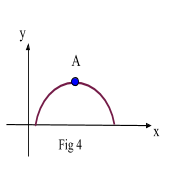2.      Minimum Point

In Fig 5 , before the stationary point (A), the gradient$\frac { dy }{ dx }$  is negative and immediately after the stationary point (A),$\frac { dy }{ dx }$  is positive . Hence, A is a minimum point.3.      Point of Inflexion

In Fig 6, points A and B are neither maximum nor minimum. Although$\frac { dy }{ dx } \quad =\quad 0$  at both the points, but it does not change sign when moving through A and B i.e if$\frac { dy }{ dx }$  x is positive before A, it will remain positive after A as well. Similarly if$\frac { dy }{ dx }$  x is negative before B, it will remain negative after B as well. Such points are called ‘Point of inflexion’.To find the nature of a stationary point, we need to find the second derivative of the function and substitute the stationary points in the equation. If our answer is positive it means that its a minimum point and vice versa.

If we differentiate a function again after finding$\frac { dy }{ dx }$,  it is called a second derivative$\frac { { d }^{ 2 }y }{ d{ x }^{ 2 } }$.

Remember:$\frac { { d }^{ 2 }y }{ d{ x }^{ 2 } } \quad \neq \quad { (\frac { dy }{ dx } ) }^{ 2 }\quad or\quad \quad \frac { { d }^{ 3 }y }{ d{ x }^{ 3 } } \quad \neq \quad { (\frac { dy }{ dx } ) }^{ 3 }$

#### Example #3

Q. The equation of a curve is$y\quad =\quad { (2x\quad -\quad 3) }^{ 2 }\quad -\quad 6x$

i) Express$\frac { dy }{ dx }$  and$\frac { { d }^{ 2 }y }{ d{ x }^{ 2 } }$  in terms of x.

ii) Find the coordinates of the two stationary points and determine the nature of each stationary point.

Solution:

i)$y\quad =\quad { (2x\quad -\quad 3) }^{ 2 }\quad -\quad 6x$$\frac { dy }{ dx } \quad =\quad { 3{ (2x\quad -\quad 3) }^{ 2 } }(2)\quad -\quad 6$$\frac { dy }{ dx } \quad =\quad 6{ (2x\quad -\quad 3) }^{ 2 }\quad -\quad 6\quad \quad \quad \quad \quad (1)$$\frac { { d }^{ 2 }y }{ d{ x }^{ 2 } } \quad =\quad 6\left[ 2(2x\quad -\quad 3)(2) \right] \quad -\quad 0$$\frac { { d }^{ 2 }y }{ d{ x }^{ 2 } } \quad =\quad 24(2x\quad -\quad 3)\quad \quad \quad \quad \quad (2)$

ii) Equate$\frac { dy }{ dx }$ to 0$6{ (2x\quad -\quad 3) }^{ 2 }\quad -\quad 6\quad =\quad 0$${ (2x\quad -\quad 3) }^{ 2 }\quad =\quad 1$${ 2x\quad -\quad 3 }\quad =\quad \pm \quad 1$

Hence:$x\quad =\quad 2,\quad 1$

When: x = 2$\frac { { d }^{ 2 }y }{ d{ x }^{ 2 } } \quad =\quad 24(2x\quad -\quad 3)$$=\quad 24(2(2)\quad -\quad 3)$$=\quad 24$

Since$\frac { { d }^{ 2 }y }{ d{ x }^{ 2 } } \quad >\quad 0$x = 2 is a minimum point.

When: x = 1$\frac { { d }^{ 2 }y }{ d{ x }^{ 2 } } \quad =\quad 24(2x\quad -\quad 3)$$=\quad 24(2(1)\quad -\quad 3)$$=\quad -24$

Since$\frac { { d }^{ 2 }y }{ d{ x }^{ 2 } } \quad <\quad 0$,  x = 1 is a maximum point.